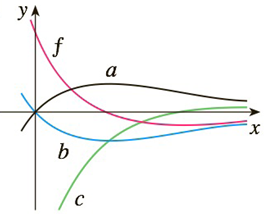Chapter 3.9, Problem 46E

Chapter
Section
Textbook Problem

# 45-46 The graph of a function f is shown. Which graph is an antiderivative of f and why?To determine

To identify:

The graph of antiderivative of f(x)

Explanation

Explanations:

1) Concept:

Use the concept of increasing and decreasing to decide the graph.

2) Given:

f(x) cuts x-axis at this point. f(x) changes its sign from positive to negative,

3) Calculation:

When the graph f

### Still sussing out bartleby?

Check out a sample textbook solution.

See a sample solution

#### The Solution to Your Study Problems

Bartleby provides explanations to thousands of textbook problems written by our experts, many with advanced degrees!

Get Started